# NCERT Exemplar Class 9 Maths Solutions for Chapter 3 - Coordinate Geometry

## NCERT Exemplar Solutions Class 9 Maths Chapter 3 – Free PDF Download

NCERT Exemplar Class 9 Maths Chapter 3 Coordinate Geometry, are provided here in the PDF format for students to prepare for exams. Students can make use of this exemplar solutions as a reference tool while practicing the NCERT textbook exercise questions, also. The exemplar problems with solutions are designed by our experts in accordance with CBSE Syllabus for 9th Standard, which covers the following topics of chapter Coordinate Geometry given below.

• Cartesian system and coordinates of the points
• Plotting a point in XY plane, if its coordinates are given
• Horizontal axis and the vertical axis
• The intersection point of the axes, etc.

Coordinate Geometry is one of the most important chapters of CBSE Class 9. In this chapter, the students will learn about the Cartesian System and plotting a point in the plane if its coordinates are given. Solve NCERT Exemplar for Class 9 Maths Chapter 3 coordinate geometry to understand the fundamentals in a better way. To promote easy learning and help students understand the concepts of coordinate geometry, free NCERT Exemplars are provided here which can be further downloaded in the form of a PDF.

## Download PDF of NCERT Exemplar Solutions for Class 9 Maths Chapter 3 – Coordinate Geometry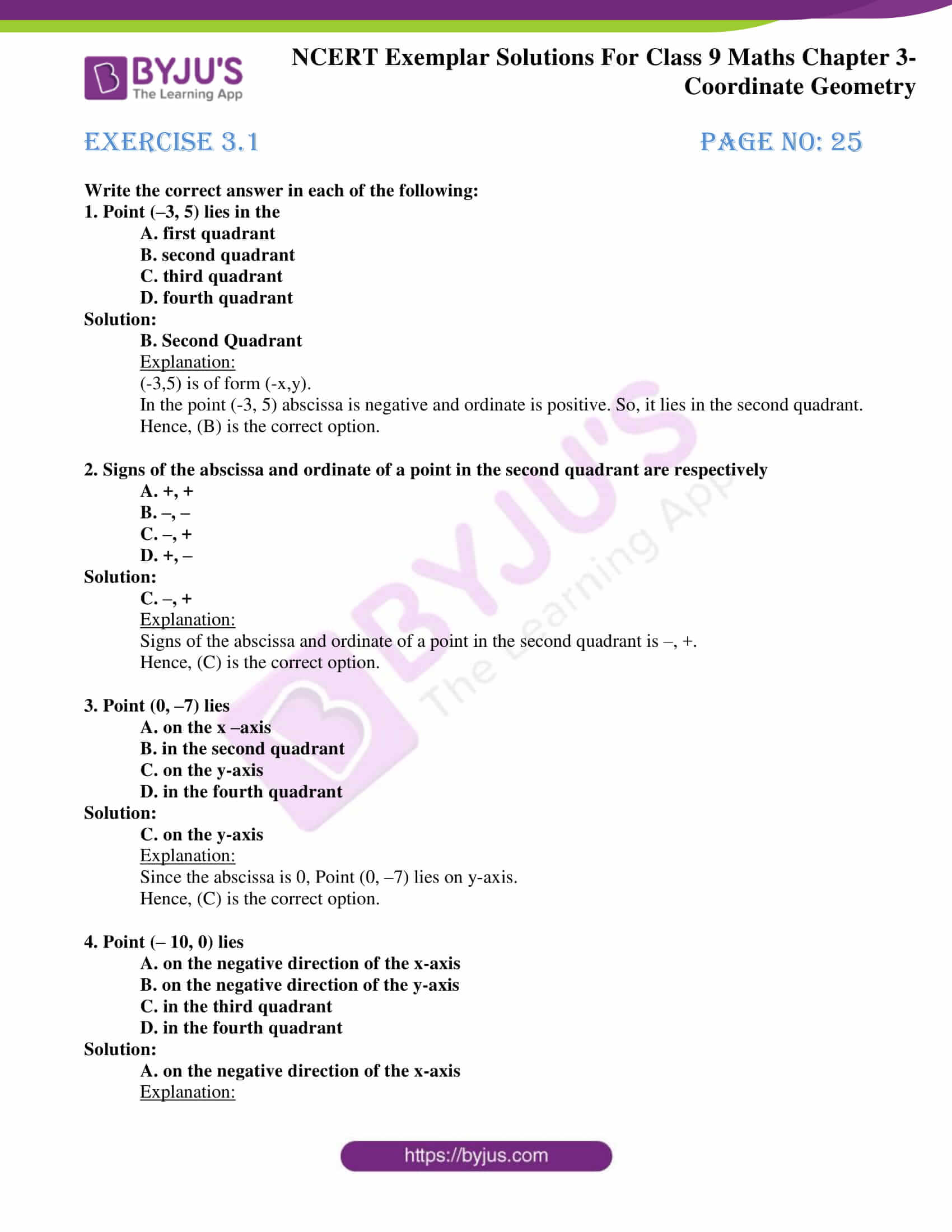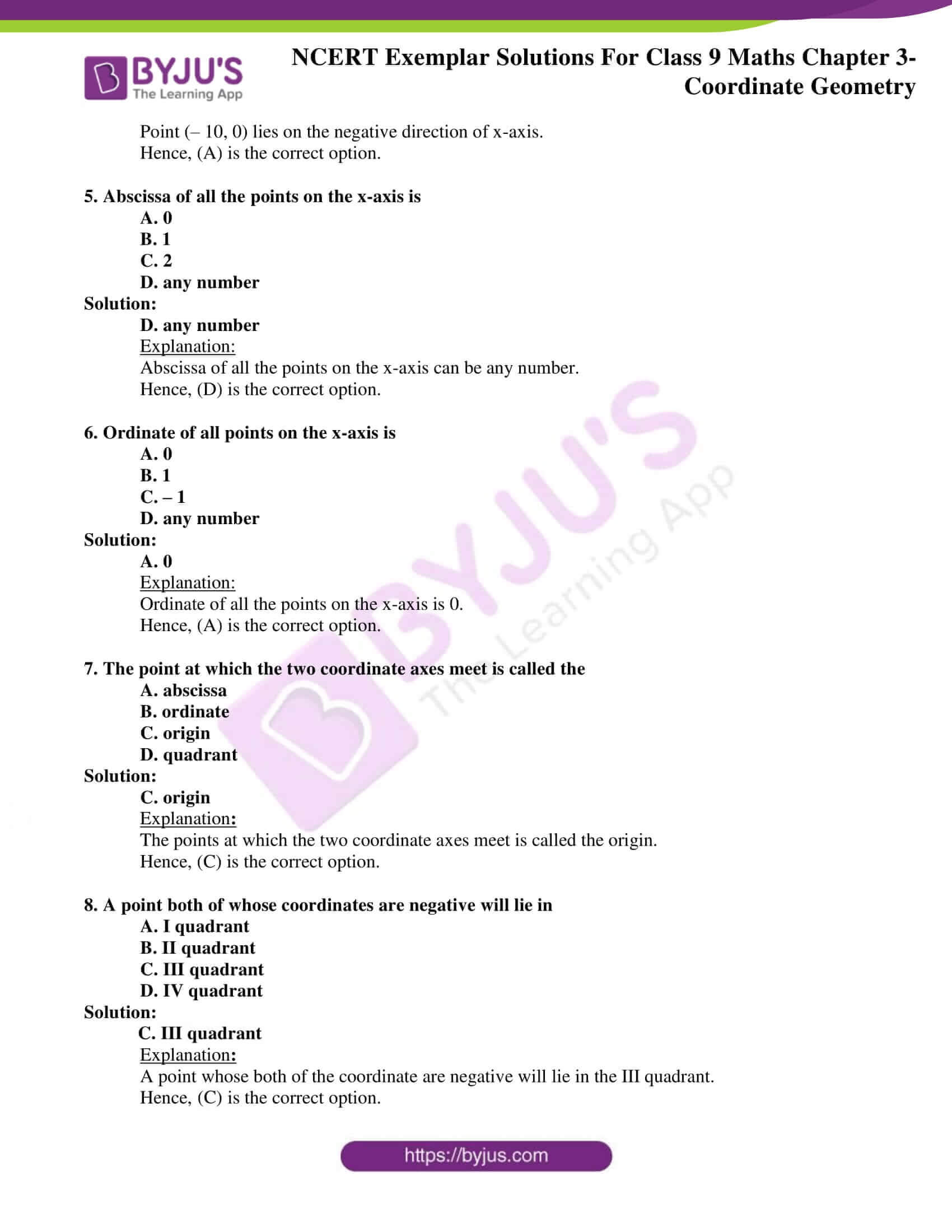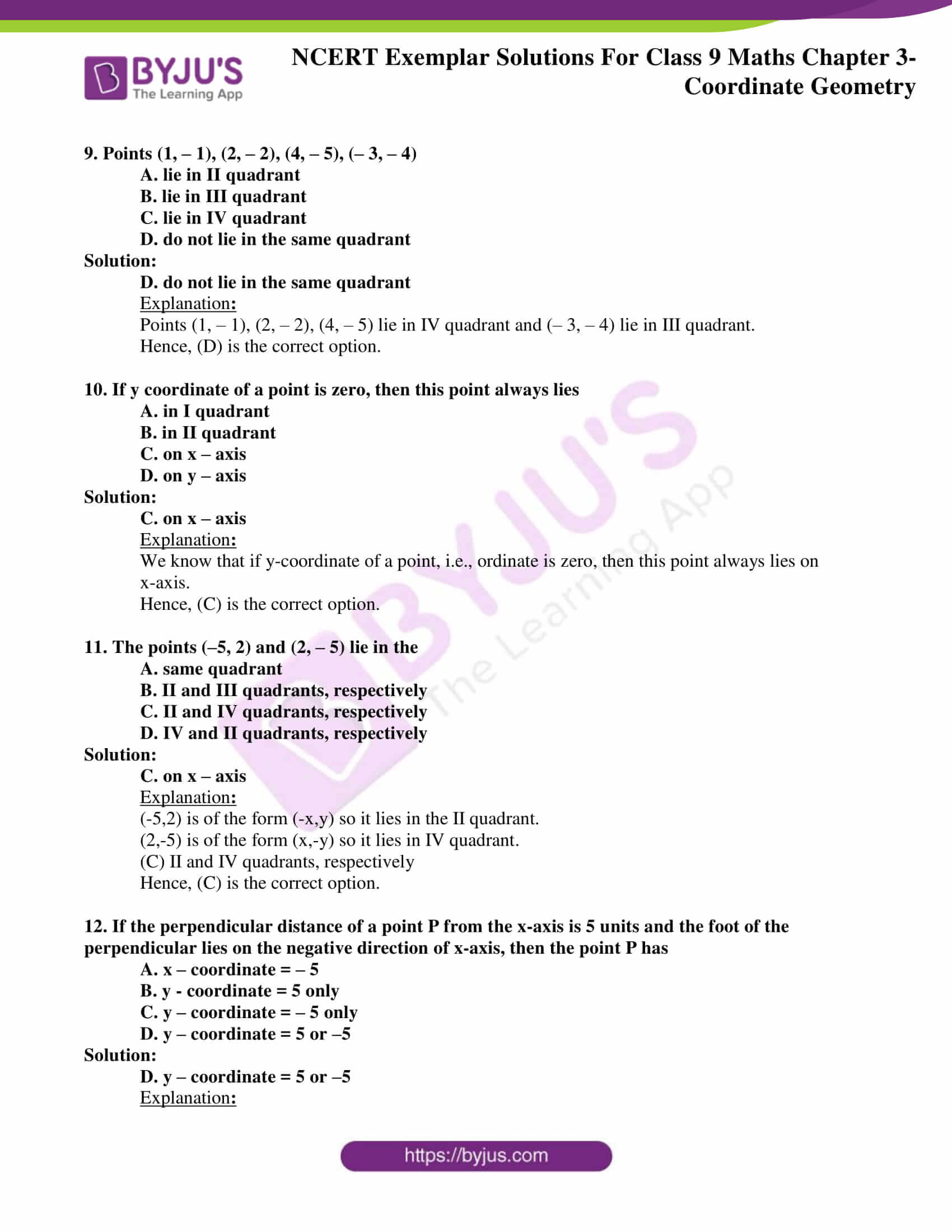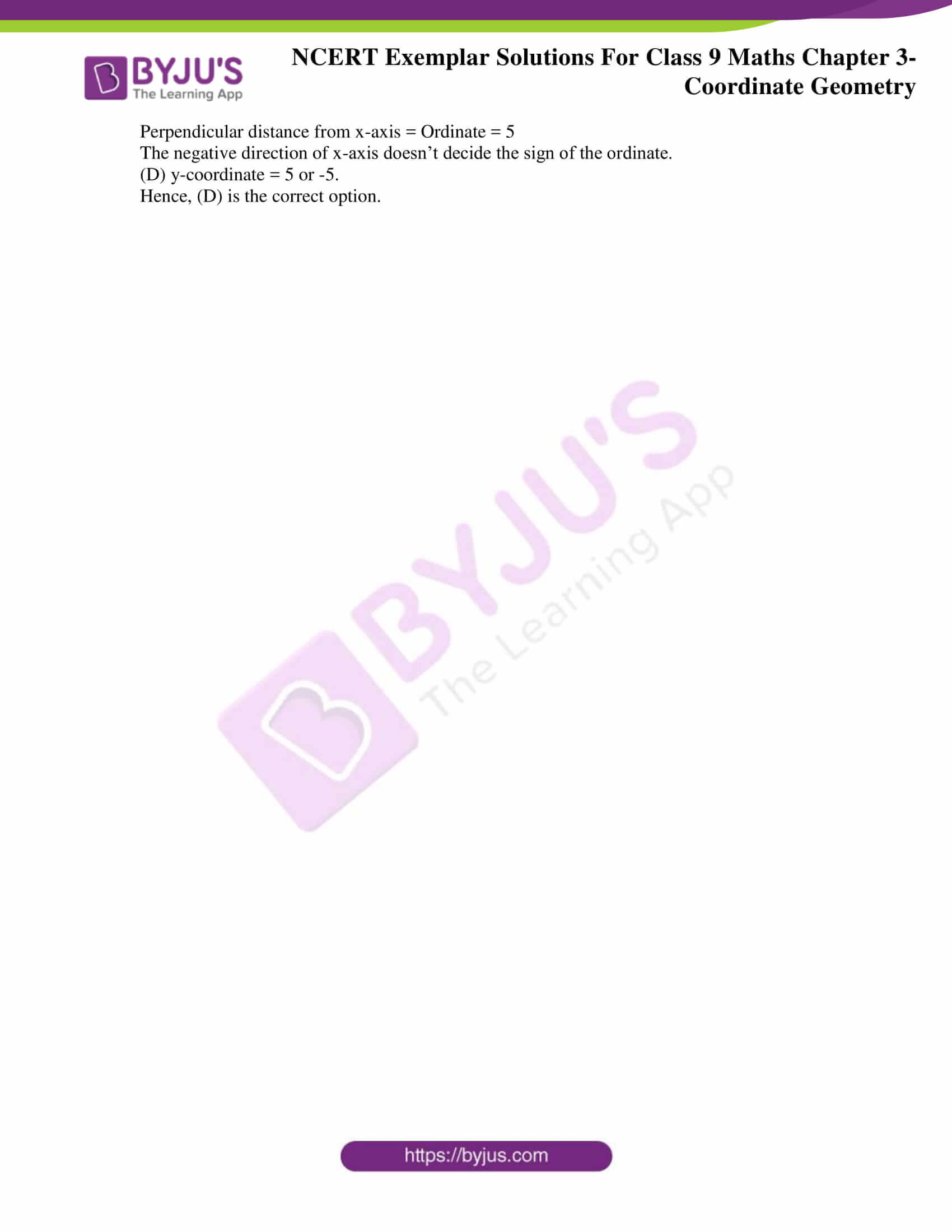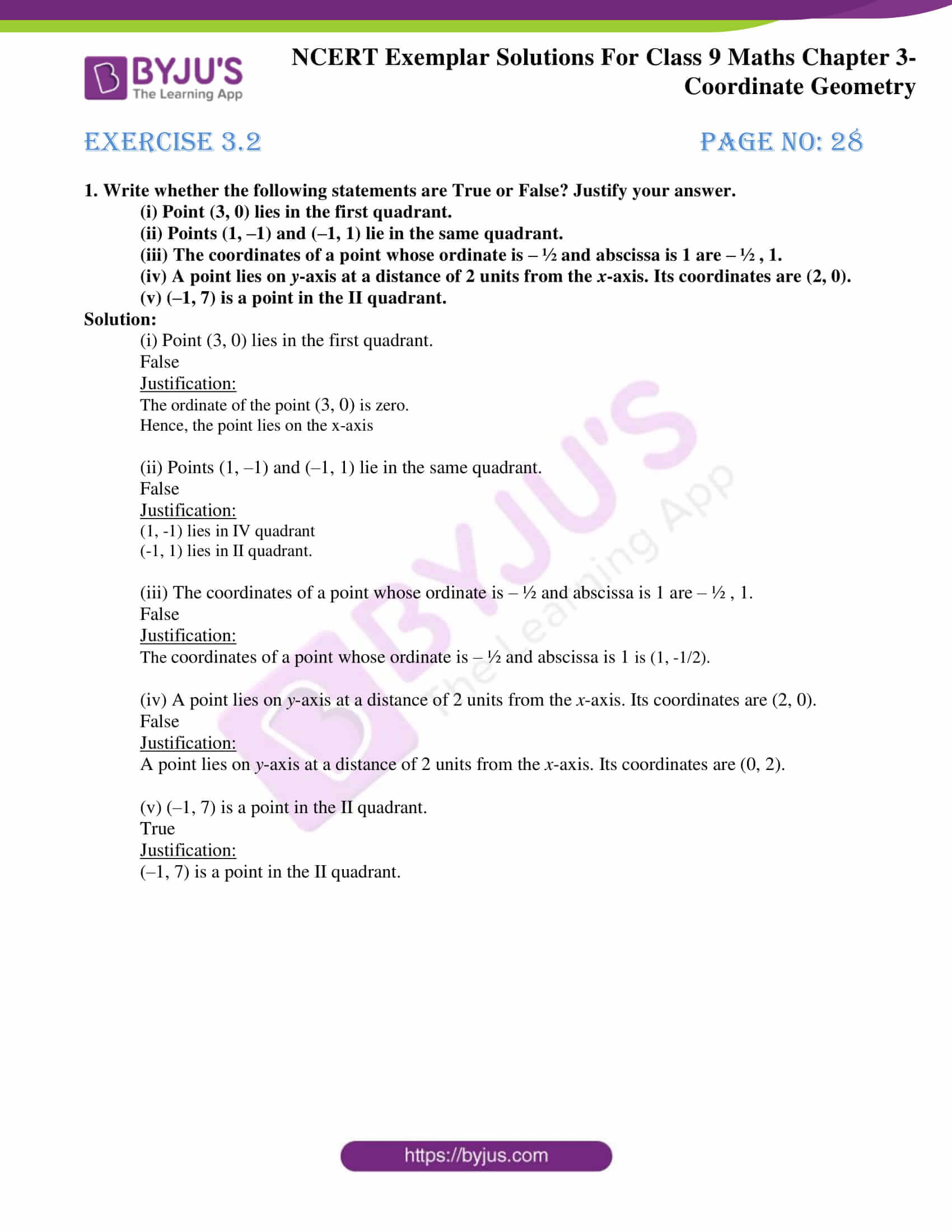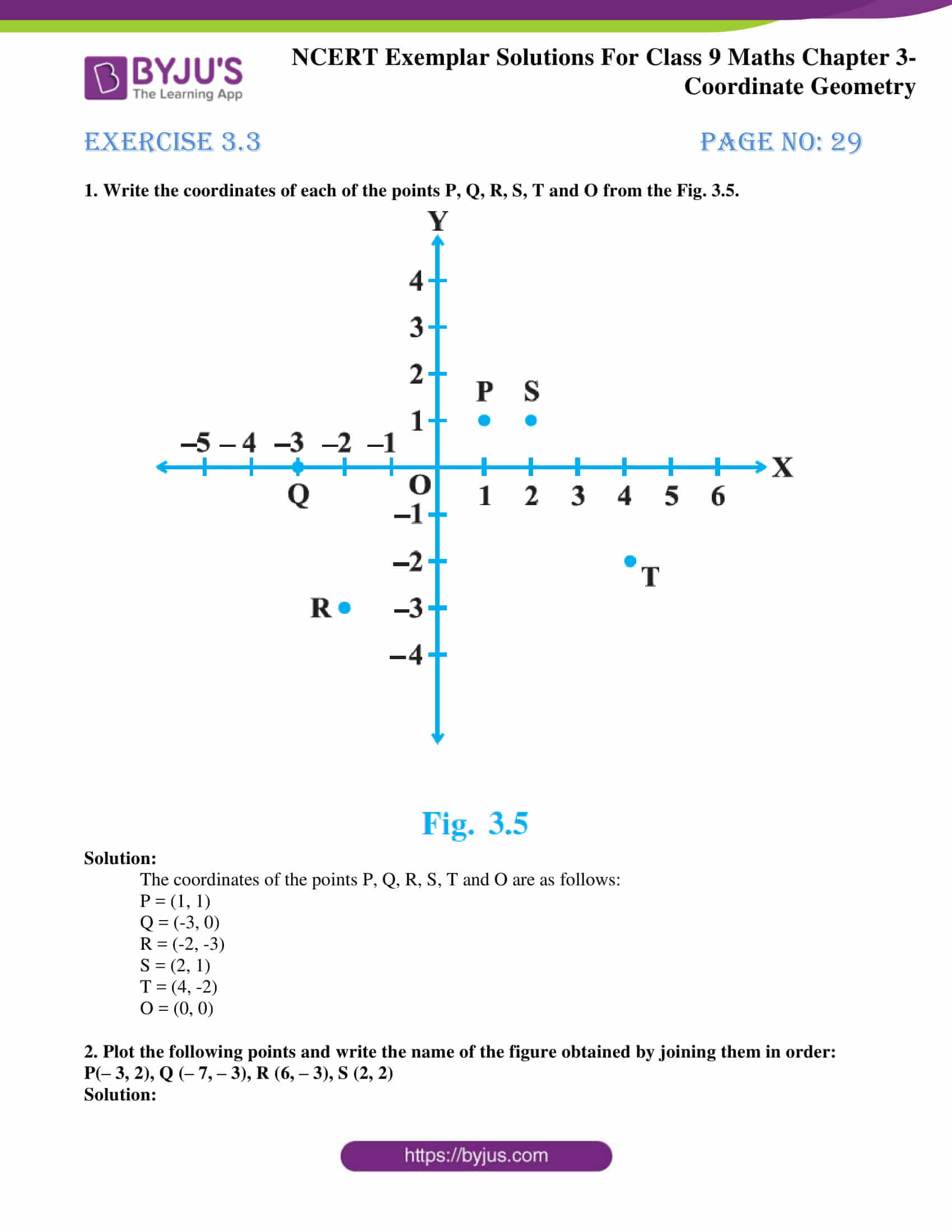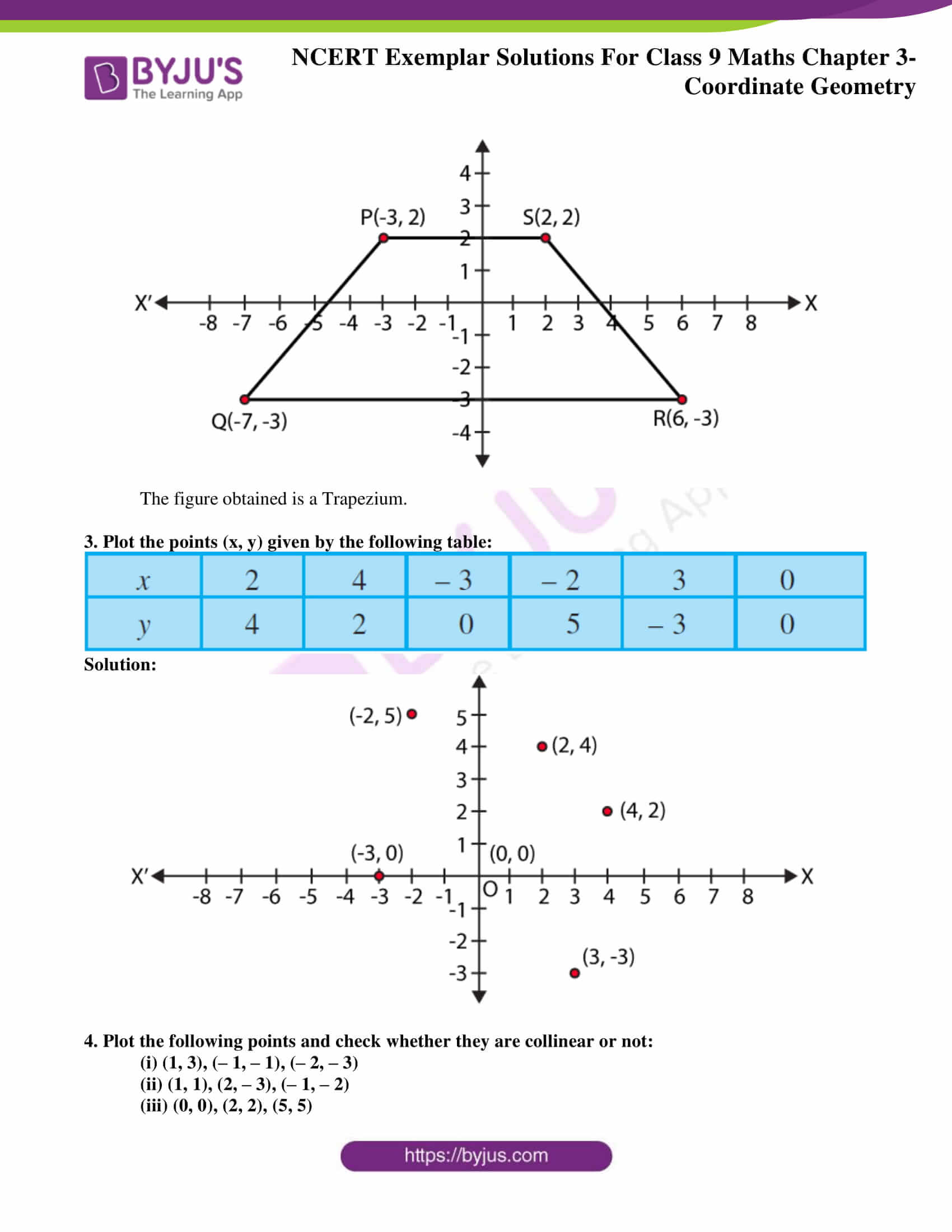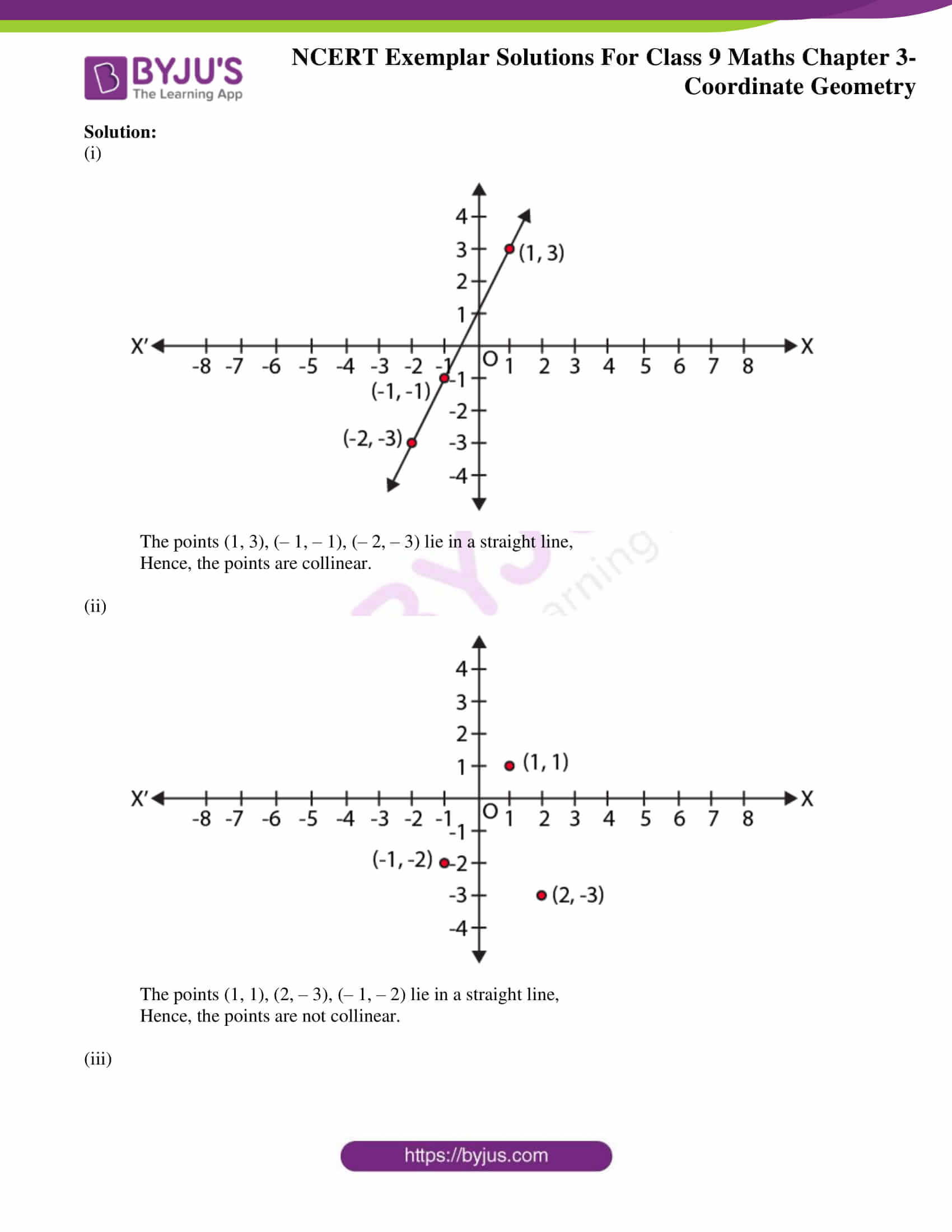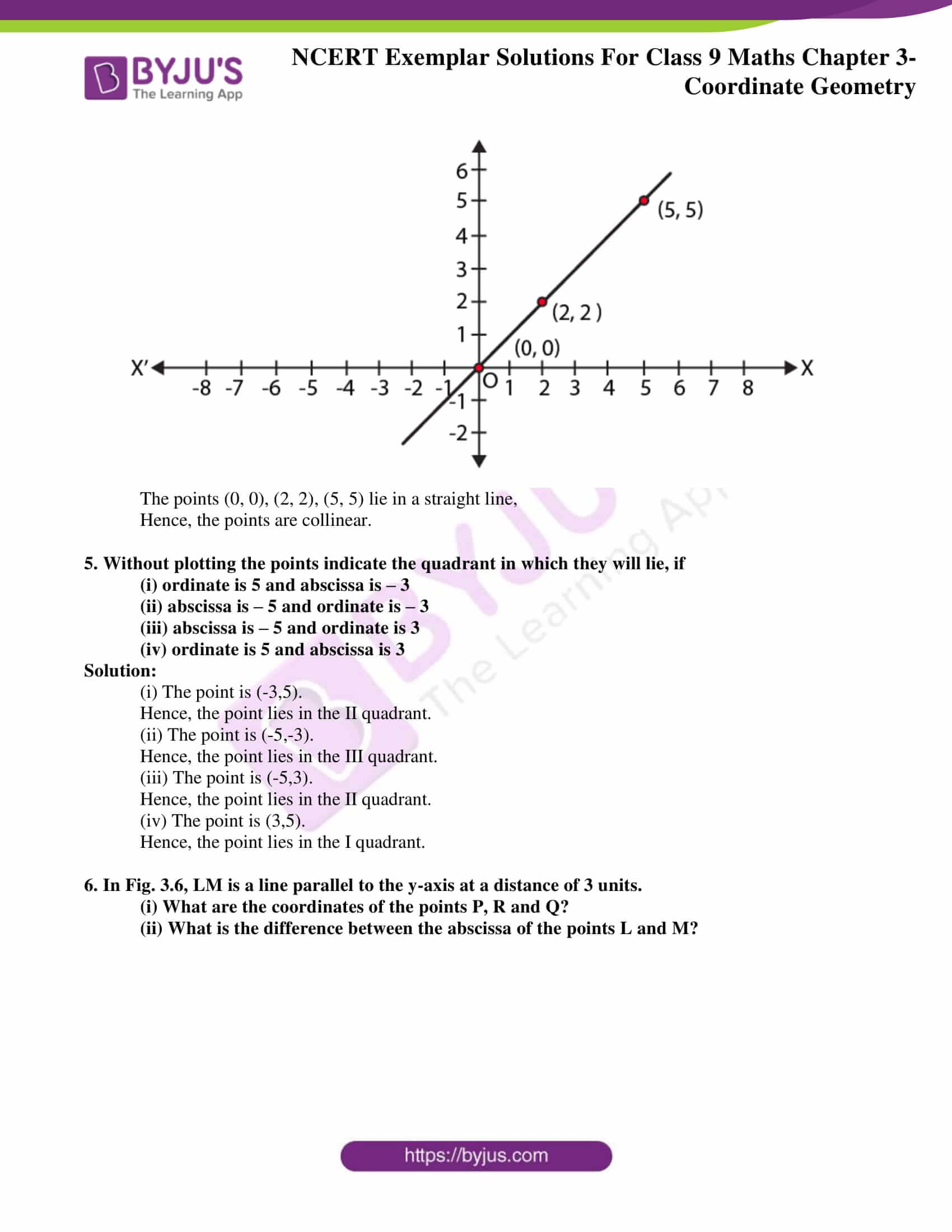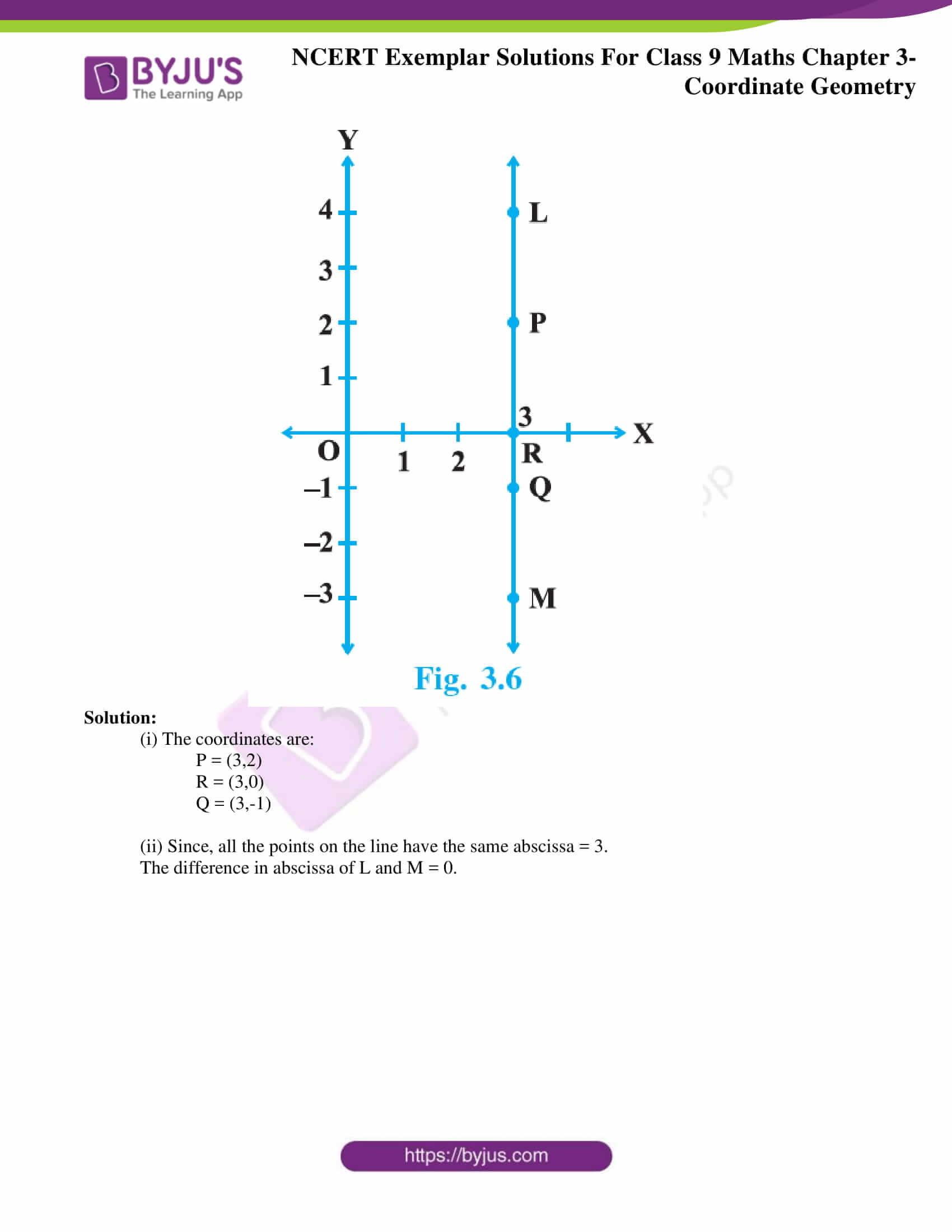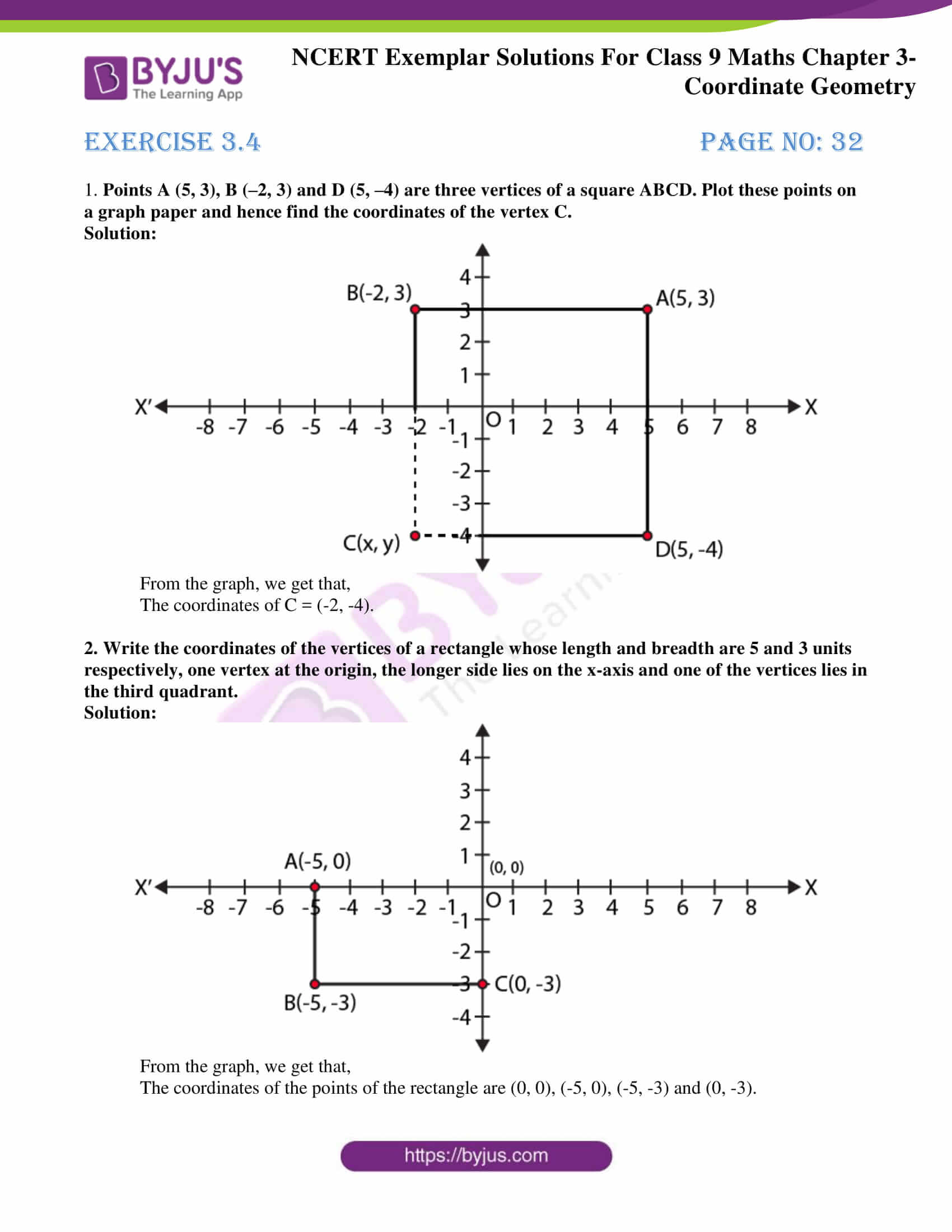### Access Answers to NCERT Exemplar Solutions for Class 9 Maths Chapter 3 Coordinate Geometry

Exercise 3.1 Page No: 25

Write the correct answer in each of the following:

1. Point (–3, 5) lies in the

Solution:

Explanation:

(-3,5) is of form (-x,y).

In the point (-3, 5) abscissa is negative and ordinate is positive. So, it lies in the second quadrant.

Hence, (B) is the correct option.

2. Signs of the abscissa and ordinate of a point in the second quadrant are respectively

A. +, +
B. –, –
C. –, +
D. +, –

Solution:

C. –, +

Explanation:

Signs of the abscissa and ordinate of a point in the second quadrant is –, +.

Hence, (C) is the correct option.

3. Point (0, –7) lies

A. on the x –axis
C. on the y-axis

Solution:

C. on the y-axis

Explanation:

Since the abscissa is 0, Point (0, –7) lies on y-axis.

Hence, (C) is the correct option.

4. Point (– 10, 0) lies

A. on the negative direction of the x-axis
B. on the negative direction of the y-axis

Solution:

A. on the negative direction of the x-axis

Explanation:

Point (– 10, 0) lies on the negative direction of x-axis.

Hence, (A) is the correct option.

5. Abscissa of all the points on the x-axis is

A. 0
B. 1
C. 2
D. any number

Solution:

D. any number

Explanation:

Abscissa of all the points on the x-axis can be any number.

Hence, (D) is the correct option.

6. Ordinate of all points on the x-axis is

A. 0
B. 1
C. – 1
D. any number

Solution:

A. 0

Explanation:

Ordinate of all the points on the x-axis is 0.

Hence, (A) is the correct option.

7. The point at which the two coordinate axes meet is called the

A. abscissa
B. ordinate
C. origin

Solution:

C. origin

Explanation:

The points at which the two coordinate axes meet is called the origin.

Hence, (C) is the correct option.

8. A point both of whose coordinates are negative will lie in

Solution:

Explanation:

A point whose both of the coordinate are negative will lie in the III quadrant.

Hence, (C) is the correct option.

9. Points (1, – 1), (2, – 2), (4, – 5), (– 3, – 4)

D. do not lie in the same quadrant

Solution:

D. do not lie in the same quadrant

Explanation:

Points (1, – 1), (2, – 2), (4, – 5) lie in IV quadrant and (– 3, – 4) lie in III quadrant.

Hence, (D) is the correct option.

10. If y coordinate of a point is zero, then this point always lies

C. on x – axis

D. on y – axis

Solution:

C. on x – axis

Explanation:

We know that if y-coordinate of a point, i.e., ordinate is zero, then this point always lies on

x-axis.

Hence, (C) is the correct option.

11. The points (–5, 2) and (2, – 5) lie in the

B. II and III quadrants, respectively
C. II and IV quadrants, respectively
D. IV and II quadrants, respectively

Solution:

C. on x – axis

Explanation:

(-5,2) is of the form (-x,y) so it lies in the II quadrant.

(2,-5) is of the form (x,-y) so it lies in IV quadrant.

(C) II and IV quadrants, respectively

Hence, (C) is the correct option.

12. If the perpendicular distance of a point P from the x-axis is 5 units and the foot of the perpendicular lies on the negative direction of x-axis, then the point P has

A. x – coordinate = – 5
B. y – coordinate = 5 only
C. y – coordinate = – 5 only
D. y – coordinate = 5 or –5

Solution:

D. y – coordinate = 5 or –5

Explanation:

Perpendicular distance from x-axis = Ordinate = 5

The negative direction of x-axis doesn’t decide the sign of the ordinate.

(D) y-coordinate = 5 or -5.

Hence, (D) is the correct option.

Exercise 3.2 Page No: 28

1. Write whether the following statements are True or False? Justify your answer.

(i) Point (3, 0) lies in the first quadrant.

(ii) Points (1, –1) and (–1, 1) lie in the same quadrant.

(iii) The coordinates of a point whose ordinate is – ½ and abscissa is 1 are – ½ , 1.

(iv) A point lies on y-axis at a distance of 2 units from the x-axis. Its coordinates are (2, 0).

(v) (–1, 7) is a point in the II quadrant.

Solution:

(i) Point (3, 0) lies in the first quadrant.

False

Justification:

The ordinate of the point (3, 0) is zero.

Hence, the point lies on the x-axis

(ii) Points (1, –1) and (–1, 1) lie in the same quadrant.

False

Justification:

(1, -1) lies in IV quadrant

(-1, 1) lies in II quadrant.

(iii) The coordinates of a point whose ordinate is – ½ and abscissa is 1 are – ½ , 1.

False

Justification:

The coordinates of a point whose ordinate is – ½ and abscissa is 1 is (1, -1/2).

(iv) A point lies on y-axis at a distance of 2 units from the x-axis. Its coordinates are (2, 0).

False

Justification:

A point lies on y-axis at a distance of 2 units from the x-axis. Its coordinates are (0, 2).

(v) (–1, 7) is a point in the II quadrant.

True

Justification:

(–1, 7) is a point in the II quadrant.

Exercise 3.3 Page No: 29

1. Write the coordinates of each of the points P, Q, R, S, T and O from the Fig. 3.5.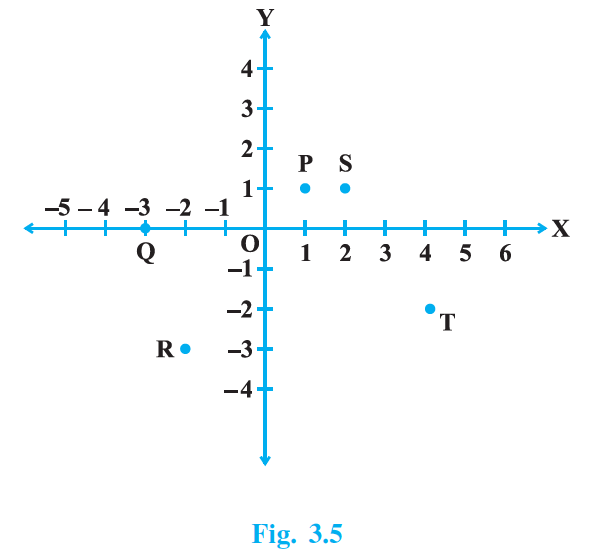Solution:

The coordinates of the points P, Q, R, S, T and O are as follows:

P = (1, 1)

Q = (-3, 0)

R = (-2, -3)

S = (2, 1)

T = (4, -2)

O = (0, 0)

2. Plot the following points and write the name of the figure obtained by joining them in order:
P(– 3, 2), Q (– 7, – 3), R (6, – 3), S (2, 2)

Solution: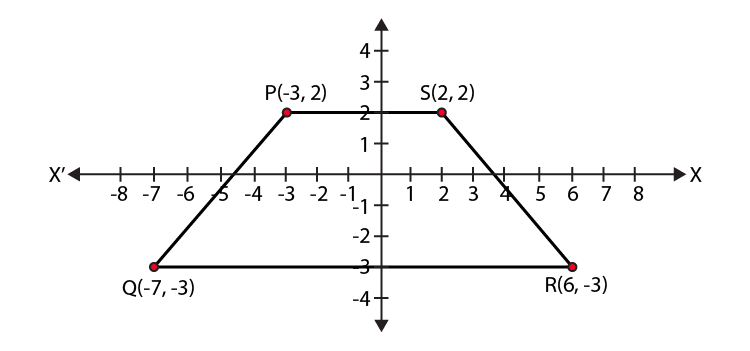The figure obtained is a Trapezium.

3. Plot the points (x, y) given by the following table: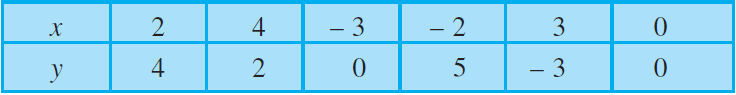Solution: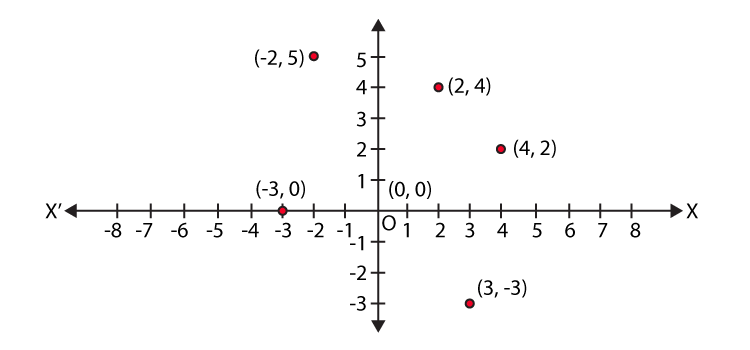4. Plot the following points and check whether they are collinear or not:

(i) (1, 3), (– 1, – 1), (– 2, – 3)

(ii) (1, 1), (2, – 3), (– 1, – 2)

(iii) (0, 0), (2, 2), (5, 5)

Solution:

(i)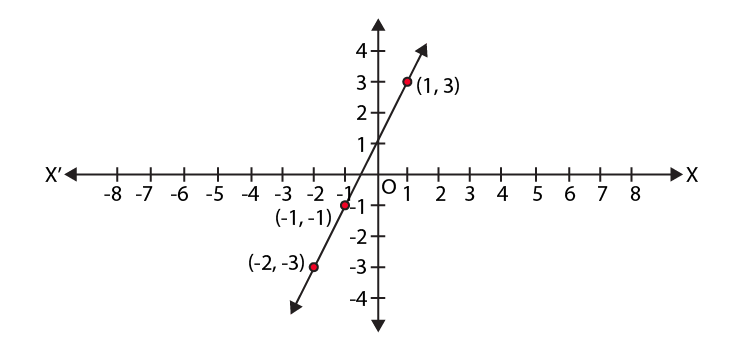The points (1, 3), (– 1, – 1), (– 2, – 3) lie in a straight line,

Hence, the points are collinear.

(ii)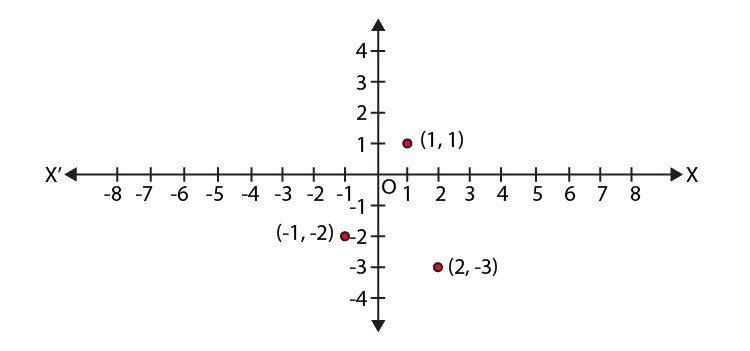The points (1, 1), (2, – 3), (– 1, – 2) lie in a straight line,

Hence, the points are not collinear.

(iii)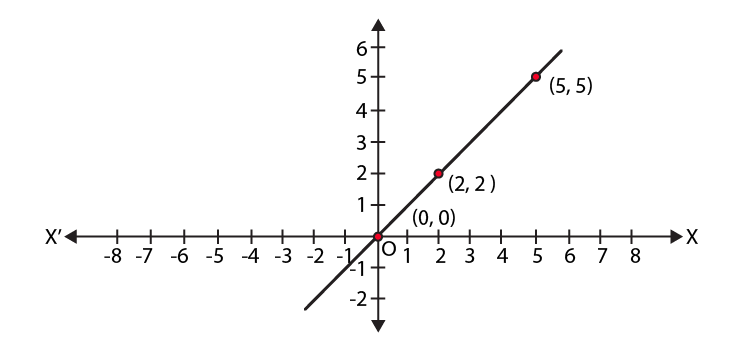The points (0, 0), (2, 2), (5, 5) lie in a straight line,

Hence, the points are collinear.

5. Without plotting the points indicate the quadrant in which they will lie, if

(i) ordinate is 5 and abscissa is – 3
(ii) abscissa is – 5 and ordinate is – 3
(iii) abscissa is – 5 and ordinate is 3
(iv) ordinate is 5 and abscissa is 3

Solution:

(i) The point is (-3,5).

Hence, the point lies in the II quadrant.

(ii) The point is (-5,-3).

Hence, the point lies in the III quadrant.

(iii) The point is (-5,3).

Hence, the point lies in the II quadrant.

(iv) The point is (3,5).

Hence, the point lies in the I quadrant.

6. In Fig. 3.6, LM is a line parallel to the y-axis at a distance of 3 units.

(i) What are the coordinates of the points P, R and Q?
(ii) What is the difference between the abscissa of the points L and M?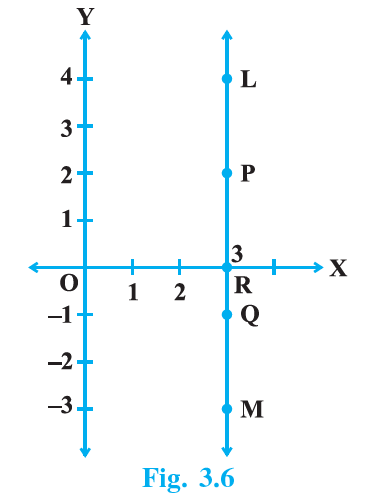Solution:

(i) The coordinates are:

P = (3,2)

R = (3,0)

Q = (3,-1)

(ii) Since, all the points on the line have the same abscissa = 3.

The difference in abscissa of L and M = 0.

Exercise 3.4 Page No: 32

1. Points A (5, 3), B (–2, 3) and D (5, –4) are three vertices of a square ABCD. Plot these points on a graph paper and hence find the coordinates of the vertex C.

Solution: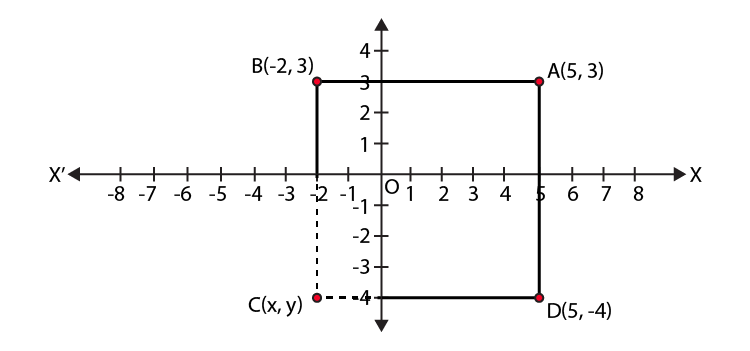From the graph, we get that,

The coordinates of C = (-2, -4).

2. Write the coordinates of the vertices of a rectangle whose length and breadth are 5 and 3 units respectively, one vertex at the origin, the longer side lies on the x-axis and one of the vertices lies in the third quadrant.

Solution: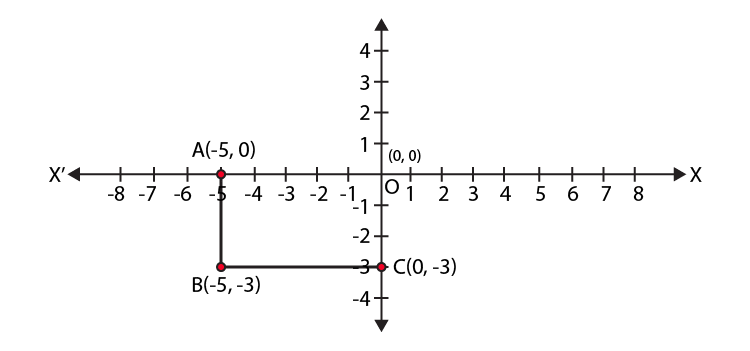From the graph, we get that,

The coordinates of the points of the rectangle are (0, 0), (-5, 0), (-5, -3) and (0, -3).

BYJU’S also provide other sources of learning such as Exemplar books, NCERT Solutions, notes and questions papers, to make students of Class 9 prepare for exams very well. Students are also advised to solve sample papers and previous year question papers to know the type of questions asked from Maths Chapter 3 and marks contained by it.

Students of the 9th standard can download BYJU’S-The Learning App to get personalized videos, explaining different types of Maths topics such as coordinate geometry and other chapter topics as well, experiencing a new way of learning to understand the concepts easily.

## Frequently Asked Questions on NCERT Exemplar Solutions for Class 9 Maths Chapter 3

### What are the topics discussed in Chapter 3 of NCERT Exemplar Solutions for Class 9 Maths as per latest CBSE syllabus?

The topics discussed in Chapter 3 of NCERT Exemplar Solutions for Class 9 Maths as per the latest CBSE syllabus are-
1.Cartesian system and coordinates of the points
2. Plotting a point in XY plane, if its coordinates are given
3. Horizontal axis and the vertical axis
4. The intersection point of the axes, etc

### Explain the Cartesian system according to Chapter 3 of NCERT Exemplar Solutions for Class 9 Maths?

A Cartesian coordinate system or Coordinate system is used to locate the position of any point and that point can be plotted as an ordered pair (x, y) known as Coordinates. The horizontal number line is called X-axis and the vertical number line is called Y-axis and the point of intersection of these two axes is known as the origin and it is denoted as ‘O’.

### Rules to Plot a Point in the Coordinate Plane in Chapter 3 of NCERT Exemplar Solutions for Class 9 Maths?

The two important rules to plot a point in the cartesian plane are given below:
1. The first coordinate in the ordered pair (x) represents the left/right movement of a point from the origin.
2. The second coordinate in the ordered pair (y) represents the up/down movement of the point from the origin.
These concepts are explained in a detailed manner to improve logical thinking abilities among students.